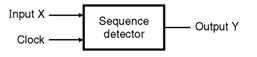Home > finite state machines > FSM Applications > Sequence Detector

# Sequence Detector

1. In the previous section we have discussed the design of a sequence generator to generate the desired sequence.
2. Now let us see how to design a sequence detector to detect a desired sequence.
3. The available sequence is applied to the input of the detector. It checks the sequence bit by bit. If required bit is at its input then the detector moves to the next state.
4. Detector output will be equal to zero as long as the complete sequence is not detected. The output will be equal to 1 if the complete sequence is detected.
5. The sequence to be detected is given to us. Follow the steps given below to design the sequence detector.Steps to design a sequence detector :

```Step 1 : 	A sequence to be detected is given to us.
Step 2 : 	Develop the state diagram.
Step 3 :	Write the state table and circuit excitation table.
Step 4 :	From the circuit excitation table write K-maps and obtain simplified equations.
Step 5 :	Draw the logic diagram.

```